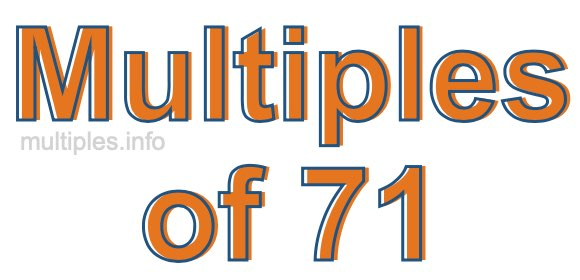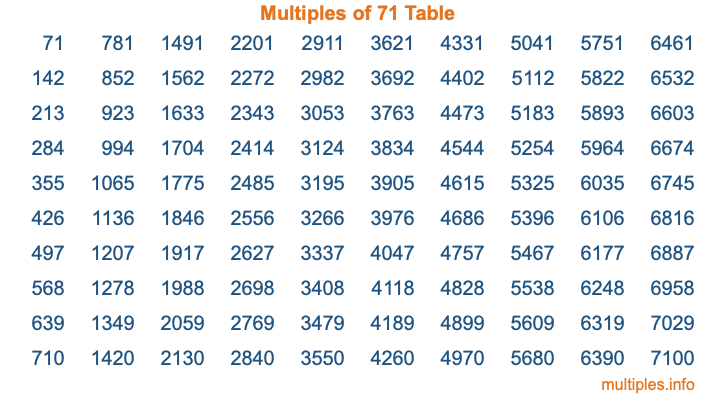Multiples of 71Welcome to the Multiples of 71 page. Here we will first teach you everything you will ever need to know about the multiples of 71, and then give you a study guide summary of everything we taught you to make sure you remember it all. Use this page to look up facts and learn information about the multiples of 71. This page will make you a multiples of seventy-one expert!

Definition of Multiples of 71
Multiples of 71 are all the numbers that when divided by 71 equal an integer. Each of the multiples of 71 are called a multiple. A multiple of 71 is created by multiplying 71 by an integer.

Therefore, to create a list of multiples of 71, you start with 1 multiplied by 71, then 2 multiplied by 71, then 3 multiplied by 71, and so on for as long as you want. Thus, the list of the first five multiples of 71 is 71, 142, 213, 284, and 355. To see a larger list of multiples of 71, see the printable image of Multiples of 71 further down on this page. We also have a category where you can choose any nth multiple of 71.

Multiples of 71 Checker
The Multiples of 71 Checker below checks to see if any number of your choice is a multiple of 71. In other words, it checks to see if there is any number (integer) that when multiplied by 71 will equal your number. To do that, we divide your number by 71. If the the quotient is an integer, then your number is a multiple of 71.

Is  a multiple of 71?

Least Common Multiple of 71 and ...
A Least Common Multiple (LCM) is the lowest multiple that two or more numbers have in common. This is also called the smallest common multiple or lowest common multiple and is useful to know when you are adding our subtracting fractions. Enter one or more numbers below (71 is already entered) to find the LCM.

Check out our LCM Calculator if you need more details about the Least Common Multiple or if you need the LCM for different numbers for adding and subtraction fractions.

nth Multiple of 71
As we stated above, 71 is the first multiple of 71, 142 is the second multiple of 71, 213 is the third multiple of 71, and so on. Enter a number below to find the nth multiple of 71.

th multiple of 71

Multiples of 71 vs Factors of 71
71 is a multiple of 71 and a factor of 71, but that is where the similarities end. All postive multiples of 71 are 71 or greater than 71. All positive factors of 71 are 71 or less than 71.

Below is the beginning list of multiples of 71 and the factors of 71 so you can compare:

Multiples of 71: 71, 142, 213, 284, 355, etc.

Factors of 71: 1, 71

As you can see, the multiples of 71 are all the numbers that you can divide by 71 to get a whole number. The factors of 71, on the other hand, are all the whole numbers that you can multiply by another whole number to get 71.

It's also interesting to note that if a number (x) is a factor of 71, then 71 will also be a multiple of that number (x).

Multiples of 71 vs Divisors of 71
The divisors of 71 are all the integers that 71 can be divided by evenly. Below is a list of the divisors of 71.

Divisors of 71: 1, 71

The interesting thing to note here is that if you take any multiple of 71 and divide it by a divisor of 71, you will see that the quotient is an integer.

Multiples of 71 Table
Below is an image of the first 100 multiples of 71 in a table. The table is in chronological order, column by column. The first column has the first ten multiples of 71, the second column has the next ten multiples of 71, and so on.The Multiples of 71 Table is also referred to as the 71 Times Table or Times Table of 71. You are welcome to print out our table for your studies.

Negative Multiples of 71
Although not often discussed or needed in math, it is worth mentioning that you can make a list of negative multiples of 71 by multiplying 71 by -1, then by -2, then by -3, and so on, to get the following list of negative multiples of 71:

-71, -142, -213, -284, -355, etc.

Multiples of 71 Summary
Below is a summary of important Multiples of 71 facts that we have discussed on this page. To retain the knowledge on this page, we recommend that you read through the summary and explain to yourself or a study partner why they hold true.

There are an infinite number of multiples of 71.

A multiple of 71 divided by 71 will equal a whole number.

71 divided by a factor of 71 equals a divisor of 71.

The nth multiple of 71 is n times 71.

The largest factor of 71 is equal to the first positive multiple of 71.

71 is a multiple of every factor of 71.

71 is a multiple of 71.

A multiple of 71 divided by a divisor of 71 equals an integer.

71 divided by a divisor of 71 equals a factor of 71.

Any integer times 71 will equal a multiple of 71.

Multiples of a Number
Here you can get the multiples of another number, all with the same attention to detail as we did for multiples of 71 on this page.

Multiples of
Multiples of 72
Did you find our page about multiples of seventy-one educational? Do you want more knowledge? Check out the multiples of the next number on our list!